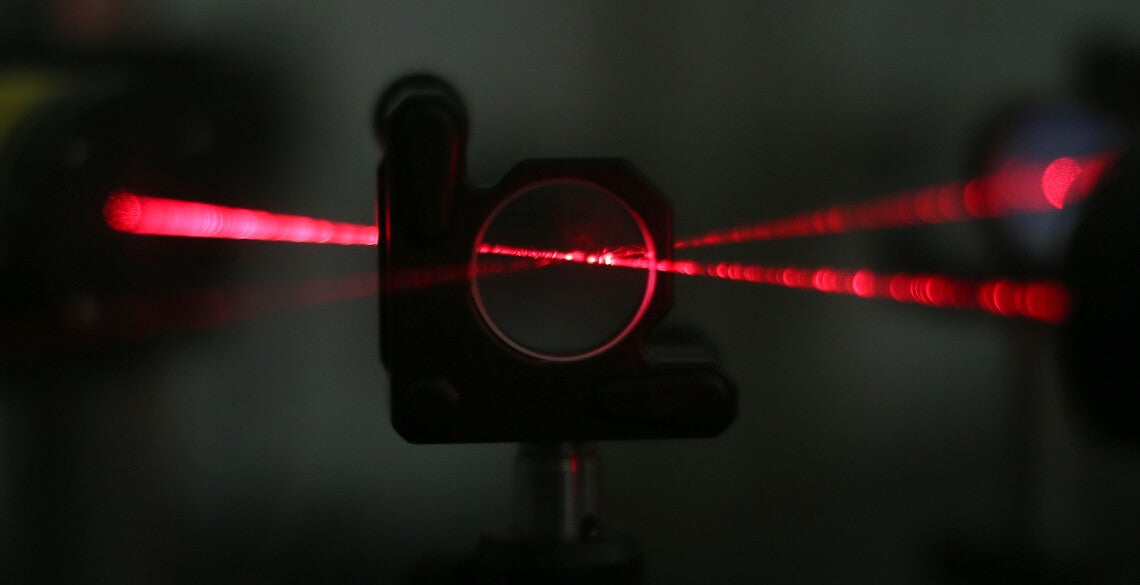# A tutorial introduction to quantum stochastic master equations based on the qubit/photon system

Published on 20 August 2022 at 15:42Pierre Rouchon I Ecole Normale Supérieure

From the key composite quantum system made of a two-level system (qubit) and a harmonic oscillator (photon) with resonant or dispersive interactions, one derives the corresponding quantum Stochastic Master Equations (SME) when either the qubits or the photons are measured. Starting with an elementary discrete-time formulation based on explicit formulae for the interaction propagators, one shows how to include measurement imperfections and decoherence. This qubit/photon quantum
system illustrates the Kraus-map structure of general discrete-time SME governing the dynamics of an open quantum system subject to measurement back-action and
decoherence induced by the environment. Then, on the qubit/photon system, one explains the passage to a continuous-time mathematical model where the measurement
signal is either a continuous real-value signal (typically homodyne or heterodyne signal) or a discontinuous and integer-value signal obtained from a counter. During this
derivation, the Kraus map formulation is preserved in an infinitesimal way. Such a derivation provides also an equivalent Kraus-map formulation to the continuous-time SME usually expressed as stochastic differential equations driven either by Wiener or Poisson processes. From such Kraus-map formulation, simple linear numerical integration scheme are derived that preserve the positivity and the trace of the density operator, i.e. of the quantum state.

Keywords: Open quantum systems, decoherence, quantum stochastic master equation, Lindblad master equation, Kraus-map, quantum channel, quantum filtering, Wiener process, Poisson process, qubit/photon composite system. Positivity and trace preserving numerical scheme. read more